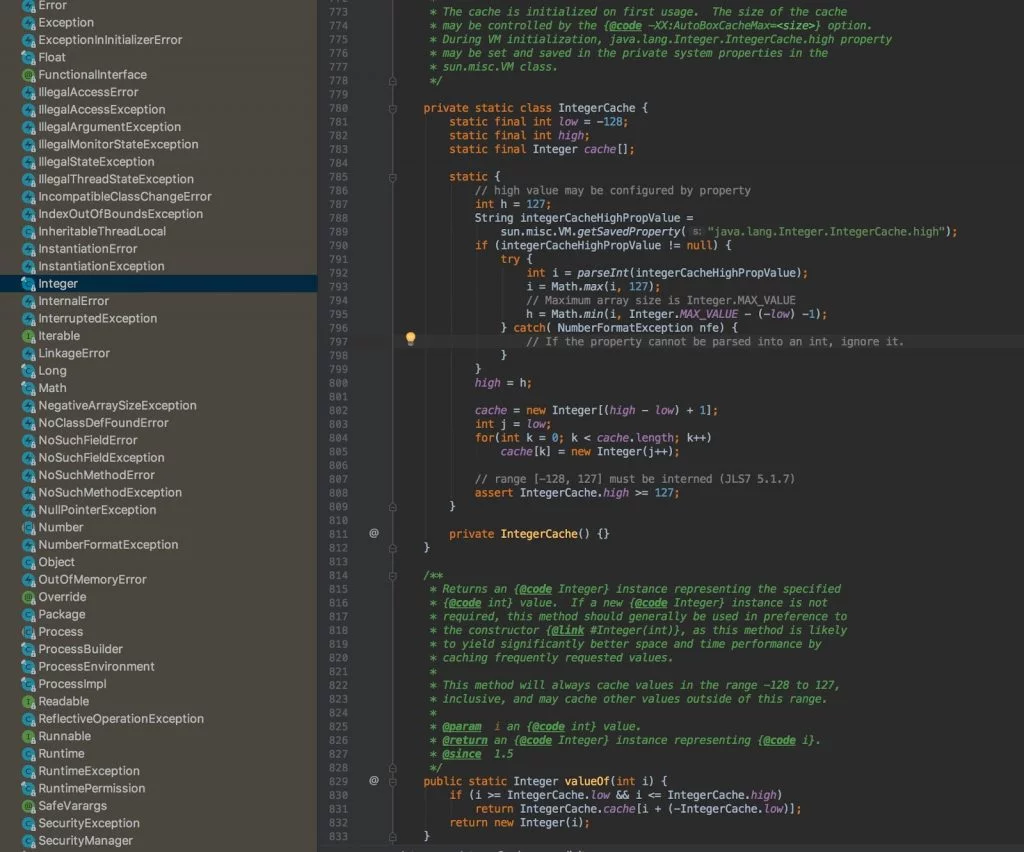# Java Integer Cache – Why Integer.valueOf(127) == Integer.valueOf(127) Is True

In an interview, one of my friends was asked that if we have two Integer objects, `Integer a = 127; Integer b = 127;` Why `a == b` evaluate to `true`when both are holding two separate objects? In this article, I will try to answer this question and also try to explain the answer.

The short answer to this question is, direct assignment of an `int` literal to an `Integer` reference is an example of auto-boxing concept where the literal value to object conversion code is handled by the compiler, so during compilation phase compiler converts `Integer a = 127;` to `Integer a = Integer.valueOf(127);`.

The`Integer` class maintains an internal IntegerCache for integers which by default ranges from `-128 to 127`and`Integer.valueOf()` method returns objects of mentioned range from that cache. So `a == b` returns true because `a`and `b` both are pointing to the same object.

In order to understand the short answer let’s first understand the Java types, all types in Java lies under two categories

1. Primitive Types: There are 8 primitive types (byte, short, int, long, float, double, char and boolean) in Java which holds their values directly in the form of binary bits.
For example `int a = 5; int b = 5;`, here `a` and `b` directly holds the binary value of 5 and if we try to compare `a` and `b` using `a == b` we are actually comparing `5 == 5` which returns true.
2. Reference Types: All types other than primitive types lies under the category of reference types e.g. Classes, Interfaces, Enums, Arrays etc. and reference types holds the address of the object instead of the object iteslf.
For example, `Integer a = new Integer(5); Integer b = new Integer(5)`, here a and b do not hold the binary value of `5`instead `a` and `b` holds memory addresses of two separate objects where both objects contain a value `5`. So if we try to compare `a` and `b` using `a == b, `we are actually comparing those two separate memory addresses hence we get `false`, to perform actual equality on `a` and `b` we need to perform `a.euqals(b)`Reference types are further divided into 4 categories Strong, Soft, Weak and Phantom References.

And we know that Java provides wrapper classes for all primitive types and support auto-boxing and auto-unboxing.

 `1` `// Example of auto-boxing, here c is a reference type`
 `2` `Integer c = ``128``; ``// Compiler converts this line to Integer c = Integer.valueOf(128);`
 `3`
 `4` `// Example of auto-unboxing, here e is a primitive type`
 `5` `int` `e = c; ``// Compiler converts this line to int e = c.intValue();`

Now if we create two integer objects`a` and`b,` and try to compare them using the equality operator`==`, we will get`false`because both references are holding different-different objects

 `Integer a = ``128``; ``// Compiler converts this line to Integer a = Integer.valueOf(128);`
 `Integer b = ``128``; ``// Compiler converts this line to Integer b = Integer.valueOf(128);`
 `System.out.println(a == b); ``// Output -- false`

But if we assign the value `127` to both `a` and `b` and try to compare them using the equality operator `==`, we will get `true` why?

 `Integer a = ``127``; ``// Compiler converts this line to Integer a = Integer.valueOf(127);`
 `Integer b = ``127``; ``// Compiler converts this line to Integer b = Integer.valueOf(127);`
 `System.out.println(a == b); ``// Output -- true`

As we can see in the code that we are assigning different objects to `a` and `b` but `a == b` can return true only if both `a`and `b` are pointing to the same object.

So how the comparison returning true? whats actually happening here? are `a` and `b` pointing to the same object?

Well till now we know that the code `Integer a = 127;` is an example of auto-boxing and compiler automatically converts this line to `Integer a = Integer.valueOf(127);`.

So it is the `Integer.valueOf()` method which is returning these integer objects which means this method must be doing something under the hood.

And if we take a look at the source code of `Integer.valueOf()` method, we can clearly see that if the passed int literal `i` is greater than `IntegerCache.low` and less than`IntegerCache.high `then the method returns Integer objects from`IntegerCache`. Default values for `IntegerCache.low` and `IntegerCache.high` are `-128` and `127` respectively.

In other words, instead of creating and retruning new integer objects, `Integer.valueOf()` method returns Integer objects from an internal `IntegerCache` if the passed int literal is greater than
`-128` and less than `127`.

 `/**`
 ` ``* Returns an {@code Integer} instance representing the specified`
 ` ``* {@code int} value.  If a new {@code Integer} instance is not`
 ` ``* required, this method should generally be used in preference to`
 ` ``* the constructor {@link #Integer(int)}, as this method is likely`
 ` ``* to yield significantly better space and time performance by`
 ` ``* caching frequently requested values.`
 ` ``*`
 ` ``* This method will always cache values in the range -128 to 127,`
 ` ``* inclusive, and may cache other values outside of this range.`
 ` ``*`
 ` ``* @param  i an {@code int} value.`
 ` ``* @return an {@code Integer} instance representing {@code i}.`
 ` ``* @since  1.5`
 ` ``*/`
 ` ``public` `static` `Integer valueOf(``int` `i) {`
 `     ``if` `(i >= IntegerCache.low && i <= IntegerCache.high)`
 `         ``return` `IntegerCache.cache[i + (-IntegerCache.low)];`
 `     ``return` `new` `Integer(i);`
 ` ``}`

Java caches integer objects which fall into -128 to 127 range because this range of integers gets used a lot in day to day programming which indirectly saves some memory.

As you can see in the following image `Integer` class maintains an inner static `IntegerCache` class which acts as the cache and holds integer objects from -128 to 127 and that’s why when we try to get integer object for `127` we always get the same object.The cache is initialized on first usage when the class get loaded into memory because of the `static block`. The max range of the cache can be controlled by the `-XX:AutoBoxCacheMax` JVM option.

This caching behavior is not applicable for `Integer` objects only, similar to `Integer.IntegerCache` we also have`ByteCache`,`ShortCache`,`LongCache`,`CharacterCache` for`Byte``Short`,
`Long`,`Character` respectively.

Byte, Short and Long have a fixed range for caching between –127 to 127 (inclusive) but for Character, the range is from 0 to 127 (inclusive). The range can be modified via argument only for Integer but not for others.

You can find the complete source code for this article on this Github Repository and please feel free to provide your valuable feedback.

## One thought on “Java Integer Cache – Why Integer.valueOf(127) == Integer.valueOf(127) Is True”

1.get private proxy says:

These are truly enormous ideas in regarding blogging.
You hve touched some good things here. Any way keep up wrinting.

Like